### Home > INT1 > Chapter Ch11 > Lesson 11.2.3 > Problem11-71

11-71.
1. Kelly is an engineer for a non-profit company that drills wells for impoverished villages in Africa. The cost of drilling a new water well is determined from the amount of drilling needed to find water, plus the fixed costs of the pump. Kelly has collected data on cost versus well depth for a sample of 12 projects: Homework Help ✎

2.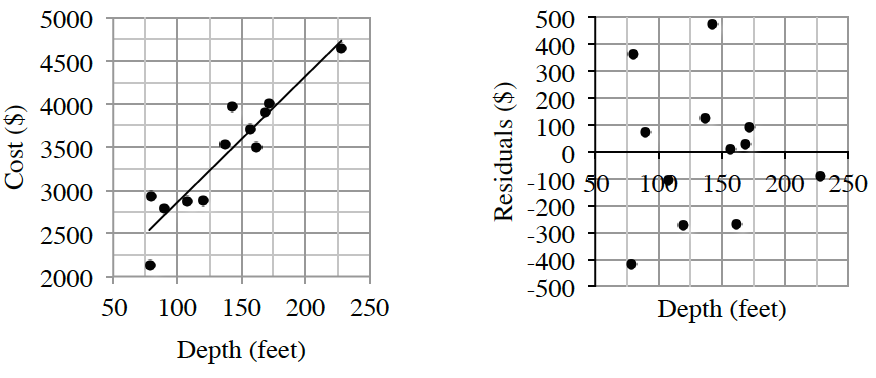1. Discuss the form, strength, direction, and outliers.

2. Interpret the slope of the least squares regression line in the context of this study.

3. Calculate and interpret R-squared in context.

4. What does the y‑intercept represent in this analysis?

5. Using the LSRL model, estimate the cost of wells that are 80, 150, and 200 feet.

6. The 80-foot well Kelly measured has a residual of $363. What was the actual cost of the well? 7. Is a linear model the best choice for predicting well cost using well depth? Support your answer.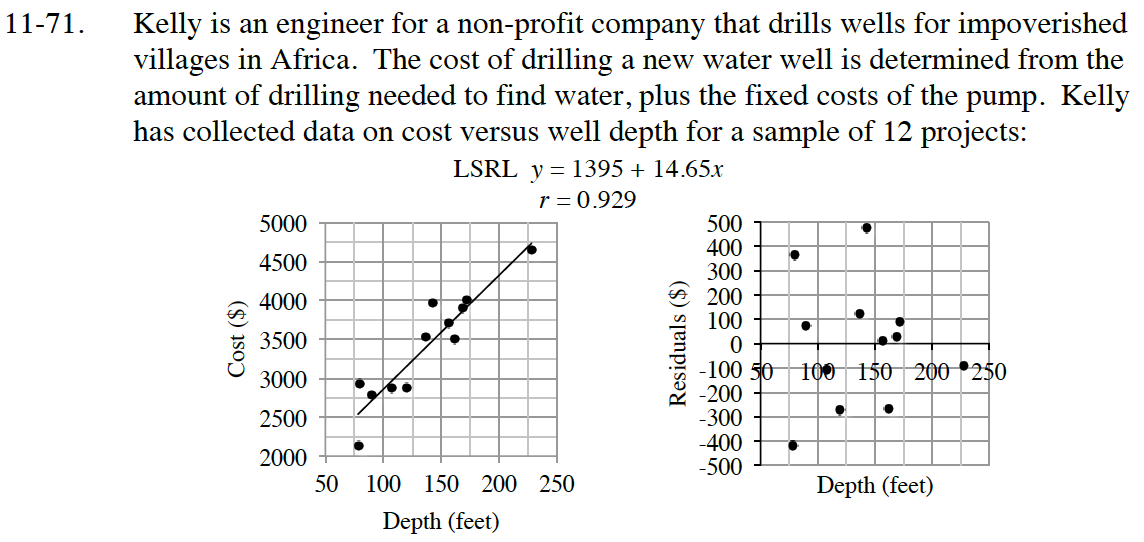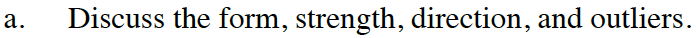Is the data in a line, a curve, or has no pattern? Does it go up, or does is go down? Do most of the point fall close to the pattern? Are there any outliers?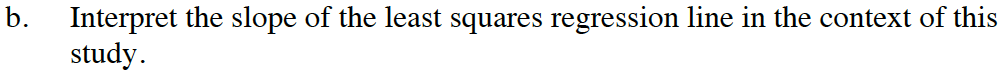$\text{Recall that slope is }\frac{\Delta y}{\Delta x}. \text{ What does the }x\text{-axis represent? What about the }y\text{-axis?}$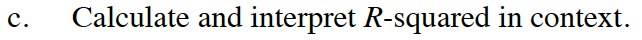R-squared: 0.864 (Note that r is given.) What does this mean in the context of the situation?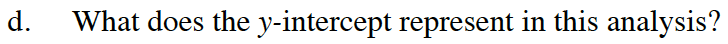The y-intercept is the point where x = 0. What is the cost to drill 0 feet?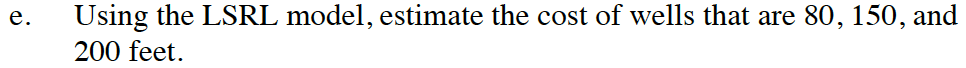Substitute 80, 150, and 200 for x in the equation of the line. 1395 + 14.65(80) =$2567
1395 + 14.65(150) = $3593 1395 + 14.65(200) =$4325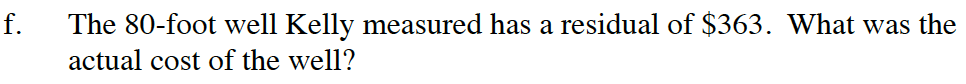Take the predicted cost of an 80 foot well, then add \$363.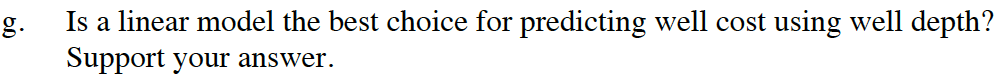Does the residual plot have a pattern? If so, another model might be better.